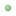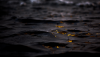Incorrect PHP?isoldehnPosts: 360 Threads: 74 Joined: Feb 2017 Reputation: 222017-11-06, 09:19 PM ``````\$countCharacters = \$db->fetch_field( \$db->query( "SELECT COUNT(*) as numberofmembers FROM ".TABLE_PREFIX."users WHERE `additionalgroups` = 8"), 'numberofmembers' ); \$countMember = \$db->fetch_field( \$db->query( "SELECT COUNT(*) as numberofmembers FROM ".TABLE_PREFIX."users WHERE `additionalgroups` = 9”), 'numberofmembers' ); \$countGameMaster = \$db->fetch_field( \$db->query( "SELECT COUNT(*) as numberofmembers FROM ".TABLE_PREFIX."users WHERE `usergroup` = 4"), 'numberofmembers' ); \$countAdmin = \$db->fetch_field( \$db->query( "SELECT COUNT(*) as numberofmembers FROM ".TABLE_PREFIX."users WHERE `usergroup` = 3"), 'numberofmembers' ); \$countCommunityHelper = \$db->fetch_field( \$db->query( "SELECT COUNT(*) as numberofmembers FROM ".TABLE_PREFIX."users WHERE `usergroup` = 6"), 'numberofmembers' ); \$countPlayers = \$countCommunityHelper + \$countAdmin + \$countGameMaster + \$countMember;`````` \$countCharacters works As soon as I add the rest the page goes blank. I have added this into the stats.php file. I’m a newbie with anything PHP related so I know this is my own error but I’ve tried following guidance of other posts here however I think I’m not understanding something.  creature-rpg.isoldehn.com > SELECT finger FROM hand WHERE id='3'Euan TDevelopment Security Web Posts: 15,170 Threads: 240 Joined: Jun 2009 Reputation: 6972017-11-06, 10:05 PM Change the code to the following: ``````\$countCharacters = \$db->fetch_field( \$db->query( "SELECT COUNT(*) as numberofmembers FROM ".TABLE_PREFIX."users WHERE `additionalgroups` = 8"), 'numberofmembers' ); \$countMember = \$db->fetch_field( \$db->query( "SELECT COUNT(*) as numberofmembers FROM ".TABLE_PREFIX."users WHERE `additionalgroups` = 9"), 'numberofmembers' ); \$countGameMaster = \$db->fetch_field( \$db->query( "SELECT COUNT(*) as numberofmembers FROM ".TABLE_PREFIX."users WHERE `usergroup` = 4"), 'numberofmembers' ); `````` The second block ("\$countMember") used a weird closing quote for the query.isoldehnPosts: 360 Threads: 74 Joined: Feb 2017 Reputation: 222017-11-06, 10:24 PM (This post was last modified: 2017-11-06, 10:40 PM by isoldehn.) Thank you! Writer error. I think I had just been staring at it for too long. (2017-11-06, 10:05 PM)Euan T Wrote: Change the code to the following: ``````\$countCharacters = \$db->fetch_field( \$db->query( "SELECT COUNT(*) as numberofmembers FROM ".TABLE_PREFIX."users WHERE `additionalgroups` = 8"), 'numberofmembers' ); \$countMember = \$db->fetch_field( \$db->query( "SELECT COUNT(*) as numberofmembers FROM ".TABLE_PREFIX."users WHERE `additionalgroups` = 9"), 'numberofmembers' ); \$countGameMaster = \$db->fetch_field( \$db->query( "SELECT COUNT(*) as numberofmembers FROM ".TABLE_PREFIX."users WHERE `usergroup` = 4"), 'numberofmembers' ); `````` The second block ("\$countMember") used a weird closing quote for the query. Also as an added question if you feel like/have the time to answer, is there a way I could simplify what I’ve done for \$countPlayers? I wasn’t sure if I could merge the counts of these: ``````\$countMember = \$db->fetch_field( \$db->query( "SELECT COUNT(*) as numberofmembers FROM ".TABLE_PREFIX."users WHERE `additionalgroups` = 9"), 'numberofmembers' ); \$countGameMaster = \$db->fetch_field( \$db->query( "SELECT COUNT(*) as numberofmembers FROM ".TABLE_PREFIX."users WHERE `usergroup` = 4"), 'numberofmembers' ); \$countAdmin = \$db->fetch_field( \$db->query( "SELECT COUNT(*) as numberofmembers FROM ".TABLE_PREFIX."users WHERE `usergroup` = 3"), 'numberofmembers' ); \$countCommunityHelper = \$db->fetch_field( \$db->query( "SELECT COUNT(*) as numberofmembers FROM ".TABLE_PREFIX."users WHERE `usergroup` = 6"), 'numberofmembers' );`````` > SELECT finger FROM hand WHERE id='3' « Next Oldest | Next Newest »

Forum Jump:

Users browsing this thread: 1 Guest(s)